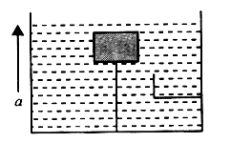Fluid statics
Question

# The tension in a string holding a solid block below the surface of a liquid (where ${\mathrm{d}}_{\mathrm{liquid}}>{\mathrm{d}}_{\mathrm{block}}$) as in shown in the figure is T when the system is at rest. Then what will be the tension in the string if the system has upward acceleration a?Easy
Solution

## here buoyant force is greater than weight of the block  $\mathrm{T}={\mathrm{vd}}_{\mathrm{l}}\mathrm{g}-{\mathrm{vd}}_{\mathrm{s}}\mathrm{g}$When it moves up with acceleration ‘a’.${\mathrm{T}}^{\text{'}}=\left({\mathrm{vd}}_{\mathrm{l}}-{\mathrm{vd}}_{\mathrm{s}}\right)\left(\mathrm{g}+\mathrm{a}\right)$$=\left({\mathrm{vd}}_{\mathrm{l}}-{\mathrm{vd}}_{\mathrm{s}}\right)\mathrm{g}\left(1+\mathrm{a}}{\mathrm{g}}\right)=\mathrm{T}\left(1+\mathrm{a}}{\mathrm{g}}\right)$

Get Instant Solutions